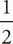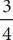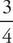# SAT Math Multiple Choice Question 84: Answer and Explanation

### Test Information

Question: 84

9.t + 4 =t - 5

In the equation above, what is the value of t ?

• A. 4
• B. 9
• C. 18
• D. 36

D Use a calculator to translate the fractions into decimals.t + 4 =t - 5 becomes 0.5t + 4 = 0.75t - 5. Subtract 0.5t from both sides to get 4 = 0.25t - 5, and then add 5 to both sides. This results in 9 = 0.25t. Use a calculator to divide! t = 36; therefore, the correct answer is (D).